Graph-Theory

 Question 1
The number of edges in a regular graph of degree d and n vertices is
 A maximum of n and d B n + d C nd D nd / 2
Engineering-Mathematics       Graph-Theory       ISRO-2018
Question 1 Explanation:
Sum of degree of vertices = 2 × no. of edges
d * n = 2 * |E|
∴ |E| = d*n/2
 Question 2
Consider the graph shown in the figure below:Which of the following is a valid strong component?
 A a, c, d B a, b, d C b, c, d D a, b, c
Engineering-Mathematics       Graph-Theory       ISRO CS 2008
Question 2 Explanation:
A directed graph is strongly connected if there is a path between all pairs of vertices. A strongly connected component (SCC) of a directed graph is a maximal strongly connected subgraph.
The graph has (a, b, c) as a strongly connected component.
 Question 3
If G is a graph with e edges and n vertices, the sum of the degrees of all vertices in G is
 A e B e/2 C e2 D 2 e
Engineering-Mathematics       Graph-Theory       ISRO CS 2009
Question 3 Explanation:

Handshaking theorem states that the sum of degrees of the vertices of a graph is twice the number of edges.

If G=(V,E) be a graph with E edges,then-

Σ degG(V) = 2E

This theorem applies even if multiple edges and loops are present.

Since the given graph is undirected, every edge contributes as twice in sum of degrees. So the sum of degrees is 2e.
 Question 4
A given connected graph is a Euler Graph if and only if all vertices of are of
 A same degree B even degree C odd degree D different degree
Engineering-Mathematics       Graph-Theory       ISRO-2016
Question 4 Explanation:
A given connected graph is a Euler Graph if and only if all vertices of are of even degree.
Proof:
→ Let G(V, E) be an Euler graph. Thus G contains an Euler line Z, which is a closed walk.
→ Let this walk start and end at the vertex u ∈ V. Since each visit of Z to an intermediate vertex v of Z contributes two to the degree of v and since Z traverses each edge exactly once, d(v) is even for every such vertex.
→ Each intermediate visit to u contributes two to the degree of u, and also the initial and ﬁnal edges of Z contribute one each to the degree of u. So the degree d(u) of u is also even.
 Question 5
The maximum number of edges in a n-node undirected graph without self loops is
 A n2 B n(n-1)/2 C n-1 D n(n+1)/2
Engineering-Mathematics       Graph-Theory       ISRO-2016
Question 5 Explanation:
The set of vertices has size n, the number of such subsets is given by the binomial coefficient C(n,2)⋅C(n,2)=n(n-1)/2.
 Question 6
A graph in which all nodes are of equal degree, is known as
 A Multigraph B Non regular graph C Regular graph D Complete graph
Engineering-Mathematics       Graph-Theory       ISRO CS 2009
Question 6 Explanation:Question 7
In a graph G there is one and only one path between every pair of vertices then G is a
 A Path B Walk C Tree D Circuit
Engineering-Mathematics       Graph-Theory       ISRO CS 2009
Question 7 Explanation:
A graph is a tree if and only if there is exactly one path between every pair of its vertices.

Let G be a graph and let there be exactly one path between every pair of vertices in G. So G is connected. Now G has no cycles, because if G contains a cycle, say between vertices u and v, then there are two distinct paths between u and v, which is a contradiction. Thus G is connected and is without cycles, therefore it is a tree.

A tree is a minimally connected graph i.e. removing a single edge will disconnect the graph. A tree with n vertices has n−1 edges and only one path exists between every pair of vertices.
 Question 8
A simple graph ( a graph without parallel edge or loops) with n vertices and k components can have at most
 A n edges B n-k edges C (n − k)(n − k + 1) edges D (n − k)(n − k + 1)/2 edges
Engineering-Mathematics       Graph-Theory       ISRO CS 2009
Question 8 Explanation:
Let G be a graph with k components. Let ni be the number of vertices in the ith component, where 1 ≤ i ≤ k. Then, the number of edges in G is equal to sum of the edges in each of its components.

So, G has maximum number of edges if each component is a complete graph.

Hence, the maximum possible number of edges in the graph G is:And in every case, (n − k)(n − k + 1)/2 will be greater than or equal to the above expression.

So, at maximum, there can be (n − k)(n − k + 1)/2 edges in a simple graph with n vertices and k components.
 Question 9
Let G be an arbitrary graph with n nodes and k components. If a vertex is removed from G, the number of components in the resultant graph must necessarily lie down between
 A k and n B k-1 and k+1 C k-1 and n-1 D k+1 and n-k
Engineering-Mathematics       Graph-Theory       ISRO CS 2009
Question 9 Explanation:
→ While a vertex is removed from a graph then that can be itself be forms a new component. The minimum number of components is k-1.
→ If a vertex is removed then it results that all the components are also be disconnected. So removal can create (n-1) components
 Question 10
How many edges are there in a forest with v vertices and k components?
 A (v+1)−k B (v+1)/2 −k C v−k D v+k
Engineering-Mathematics       Graph-theory       ISRO CS 2011
Question 10 Explanation:
Method-1:
→ Suppose, if each vertex is a component, then k=v, then there will not be any edges among them. So, v-k= 0 edges.
Method-2:
→ According to pigeonhole principle, every component have v/k vertices.
→ Every component there will be (v/k)-1 edges.
→ Total k components and edges= k*((v/k)-1)
= v–k
 Question 11
The number of edges in a ‘n’ vertex complete graph is ?
 A n * (n-1) / 2 B n * (n+1) / 2 C n2 D n * (n+1)
Engineering-Mathematics       Graph-theory       ISRO CS 2013
Question 11 Explanation:
Complete graph is an undirected graph in which each vertex is connected to every vertex, other than itself. If there are ‘n’ vertices then total edges in a complete graph is n(n-1)/2
 Question 12

To guarantee correction of upto t errors, the minimum Hamming distance dmin in a block code must be

 A t+1 B t−2 C 2t−1 D 2t+1
Engineering-Mathematics       Graph-Theory       UGC-NET JUNE Paper-2
Question 12 Explanation:
For detect t-bit errors a hamming distance of t+1 bit is needed but to correct the t-bit error the hamming distance of 2t+1 bit is needed.
 Question 13
Which of the following statements is/are TRUE for an undirected graph?
P: Number of odd degree vertices is even
Q: Sum of degrees of all vertices is even
 A P only B Q only C Both P and Q D Neither P nor Q
Engineering-Mathematics       Graph-Theory       Nielit Scientist-B CS 22-07-2017
Question 13 Explanation:
Euler’s Theorem 3:
The sum of the degrees of all the vertices of a graph is an even number (exactly twice the number of edges).
In every graph, the number of vertices of odd degree must be even.
 Question 14
Consider the following graph L and find the bridges, if any.A No bridges B {d,e} C {c,d} D {c,d} and {c,f}
Engineering-Mathematics       Graph-Theory       Nielit Scientist-B CS 22-07-2017
Question 14 Explanation:
A bridge, ut-edge, or cut arc is an edge of a graph whose deletion increases its number of connected components.
Equivalently, an edge is a bridge if and only if it is not contained in any cycle.
A graph is said to be bridgeless or isthmus-free if it contains no bridges.
If we remove {d,e} edge then there is no way to reach e and the graph is disconnected.
The removal of edges {c,d} and {c,f} makes graph disconnect but this forms a cycle.
 Question 15
The following graph nas no Euler circuit becauseA It has 7 vertices B It is even-valent(all vertices have even valence) C It is not connected D It does not have a Euler circuit
Engineering-Mathematics       Graph-Theory       Nielit Scientist-B CS 22-07-2017
Question 15 Explanation:
An Eulerian trail or Euler walk in an undirected graph is a walk that uses each edge exactly once. If such a walk exists, the graph is called traversable or semi-eulerian.
Important Properties:
→ An undirected graph has an Eulerian cycle if and only if every vertex has even degree, and all of its vertices with nonzero degree belong to a single connected component.
→ An undirected graph can be decomposed into edge-disjoint cycles if and only if all of its vertices have even degree. So, a graph has an Eulerian cycle if and only if it can be decomposed into edge-disjoint cycles and its nonzero-degree vertices belong to a single connected component.
→ An undirected graph has an Eulerian trail if and only if exactly zero or two vertices have odd degree, and all of its vertices with nonzero degree belong to a single connected component.
→ A directed graph has an Eulerian cycle if and only if every vertex has equal in degree and out degree, and all of its vertices with nonzero degree belong to a single strongly connected component. Equivalently, a directed graph has an Eulerian cycle if and only if it can be decomposed into edge-disjoint directed cycles and all of its vertices with nonzero degree belong to a single strongly connected component.
→ A directed graph has an Eulerian trail if and only if at most one vertex has (out-degree) − (in-degree) = 1, at most one vertex has (in-degree) − (out-degree) = 1, every other vertex has equal in-degree and out-degree, and all of its vertices with nonzero degree belong to a single connected component of the underlying undirected graph
 Question 16
For the graph shown, which of the following paths is a Hamilton circuit?A ABCDCFDEFAEA B AEDCBAF C AEFDCBA D AFCDEBA
Engineering-Mathematics       Graph-Theory       Nielit Scientist-B CS 22-07-2017
Question 16 Explanation:
A Hamiltonian cycle, also called a Hamiltonian circuit, Hamilton cycle, or Hamilton circuit, is a graph cycle (i.e., closed loop) through a graph that visits each node exactly once. A graph possessing a Hamiltonian cycle is said to be a Hamiltonian graph.
Here, Option A: A,F and E are repeated several times.
Option B: It is not a cycle. It means, not closed walk
Option C: It is closed walk and all vertex traversed. So this is final answer.
Option D: It’s not a closed walk.
 Question 17
If G is an undirected planar graph on n vertices with e edges then
 A e<=n B e<=2n C e<=en D None of the option
Engineering-Mathematics       Graph-Theory       Nielit Scientist-B CS 22-07-2017
Question 17 Explanation:
Euler's formula: states that if a finite, connected, planar graph is drawn in the plane without any edge intersections, and v is the number of vertices, e is the number of edges and f is the number of faces (regions bounded by edges, including the outer, infinitely large region), then
v − e + f = 2.
→ For a simple, connected, planar graph with v vertices and e edges and faces, the following simple conditions hold for v ≥ 3:
Theorem 1. e ≤ 3v − 6;
Theorem 2. If there are no cycles of length 3, then e ≤ 2v − 4.
Theorem 3. f ≤ 2v − 4.
Theorem (The handshaking theorem):
Let G be an undirected graph (or multigraph) with V vertices and N edges. ThenExercise:
Suppose a simple graph has 15 edges, 3 vertices of degree 4, and all others of degree 3. How many vertices does the graph have?
3*4+(x-3)*3=30
 Question 18
Choose the most appropriate definition of plane graph.
 A A simple graph which is isomorphic to hamiltonian graph B A graph drawn in a plane in such a way that if the vertex set of graph can be partitioned into two non-empty disjoint subset X and Y in such a way that each edge of G has one end in X and one end in Y C A graph drawn in a plane in such a way that any pair of edges meet only at their end vertices D None of the option
Engineering-Mathematics       Graph-Theory       Nielit Scientist-B CS 22-07-2017
Question 18 Explanation:
In graph theory, a planar graph is a graph that can be embedded in the plane, i.e., it can be drawn on the plane in such a way that its edges intersect only at their endpoints.
In other words, it can be drawn in such a way that no edges cross each other. Such a drawing is called a plane graph or planar embedding of the graph.
 Question 19

The K-coloring of an undirected graph G = (V, E) is a function

C: V ➝ {0, 1, ......, K-1} such that c(u)≠c(v) for every edge (u,v) ∈ E

Which of the following is not correct?

 A G has no cycles of odd length B G has cycle of odd length C G is 2-colorable D G is bipartite
Engineering-Mathematics       Graph-Theory       UGC-NET DEC Paper-2
Question 19 Explanation:
• A k-colouring of a graph G consists of k different colours and G is then called k-colourable.
• A cycle of length n ≥ 3 is 2-chromatic if n is even and 3-chromatic if n is odd.
• A graph is bi-colourable (2-chromatic) if and only if it has no odd cycles.
• A nonempty graph G is bicolourable if and only if G is bipartite
 Question 20

If a graph (G) has no loops or parallel edges and if the number of vertices(n) in the graph is n≥3, then the graph G is Hamiltonian if

(i) deg(v) ≥ n/3 for each vertex v
(ii)
deg(v) + deg(w) ≥ n whenever v and w are not connected by an edge.
(iii) E(G) ≥ 1/3(n-1)(n-2) + 2

Choose the correct answer for the code given below:

Code:
 A (i) and (iii) only B (ii) and (iii) only C (iii) only D (ii) only
Engineering-Mathematics       Graph-Theory       UGC-NET DEC Paper-2
Question 20 Explanation:
Option-2 is correct.
→ Dirac's theorem on Hamiltonian cycles, the statement that an n-vertex graph in which each vertex has degree at least n/2 must have a Hamiltonian cycle.
→ Dirac's theorem on chordal graphs, the characterization of chordal graphs as graphs in which all minimal separators are cliques.
→ Dirac's theorem on cycles in k-connected graphs, the result that for every set of k vertices in a k-vertex-connected graph there exists a cycle that passes through all the vertices in the set.
 Question 21
The number of independent loops for a network with n nodes and b branch is
 A n-1 B b-n C b-n+1 D independent of "the number of nodes
Engineering-Mathematics       Graph-Theory       NieLit STA 2016 March 2016
Question 21 Explanation:
● A loop is a closed path in a circuit. Loop counts starting at a node passing through a set of nodes and returning to the starting node without passing through any node more than once.
● A loop is said to be independent if it contains at least one branch which is not a part of any other independent loop.
● A network with ‘b’ branches, ‘n’ nodes and ‘L’ independent loops will satisfy the fundamental theorem of network topology.
b=L+n−1
L=b−n+1
 Question 22
The number of circuits in a tree with 'n' nodes is
 A Zero B One C n-1 D n/2
Engineering-Mathematics       Graph-Theory       NieLit STA 2016 March 2016
Question 22 Explanation:
● A tree (e.g. a binary tree) has a root, branches (nodes), and leaf nodes.
● A circuit in such a tree is impossible (unless you have some other data structure as well such as a linked list or you just program the tree so badly it ends up with circuits).
 Question 23
A complete graph with "n" vertices is
 A 2-chromatic B (n/2) chromatic C (n-1) chromatic D n-Chromatic
Engineering-Mathematics       Graph-Theory       NieLit STA 2016 March 2016
Question 23 Explanation:Question 24
Minimum number of colours required to colour the vertices of a cycle with n nodes in such a way that no two adjacent nodes have the same colour is
 A 2 B 3 C 4 D n-2⌈(n/2)⌉+2
Engineering-Mathematics       Graph-Theory       NieLit STA 2016 March 2016
Question 24 Explanation:
We need 3 colors to color a odd cycle and 2 colors to color an even cycle.
 Question 25
Let G be a simple undirected planar graph on 10 vertices with 15 edges. If G is a connected graph, then the number of bounded faces in any embedding of G on the plane is equal to:
 A 3 B 4 C 5 D 6
Engineering-Mathematics       Graph-Theory       Nielit Scientist-B CS 4-12-2016
Question 25 Explanation:
v - e + f = 2
‘v’ is number of vertices and ‘e’ is number of edges
‘f’ is number of faces including bounded and unbounded
10 - 15 + f = 2
f = 7
There is always one unbounded face, so the number of bounded faces = 6
 Question 26
Given an undirected graph G with V vertices and E edges, the sum of the degrees of all vertices is
 A E B 2E C V D 2V
Engineering-Mathematics       Graph-Theory       Nielit Scientist-B IT 22-07-2017
Question 26 Explanation:
Theorem (Sum of Degrees of Vertices Theorem):
Suppose a graph has n vertices with degrees d 1 , d 2 , d 3 , ..., d n.
Add together all degrees to get a new number
d 1 + d 2 + d 3 + . .. + d n = D v . Then D v = 2 e .
In words, for any graph the sum of the degrees of the vertices equals twice the number of edges.
 Question 27
A path in graph G, which contains every vertex of G and only Once?
 A Euler circuit B Hamiltonian path C Euler Path D Hamiltonian Circuit
Engineering-Mathematics       Graph-Theory       Nielit Scientist-B IT 22-07-2017
Question 27 Explanation:
● Hamiltonian path, also called a Hamilton path, is a graph path between two vertices of a graph that visits each vertex exactly once. If a Hamiltonian path exists whose endpoints are adjacent, then the resulting graph cycle is called a Hamiltonian cycle (or Hamiltonian cycle)
● An Euler path is a path that uses every edge of a graph exactly once. An Euler circuit is a circuit that uses every edge of a graph exactly once.
● An Euler path starts and ends at different vertices.
● An Euler circuit starts and ends at the same vertex.
 Question 28
Considering the following graph, which one of the following set of edged represents all the bridges of the given graph?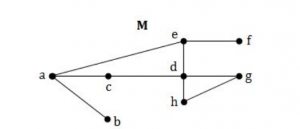A (a,b)(e,f) B (a,b),(a,c) C (c,d)(d,h) D (a,b)
Engineering-Mathematics       Graph-Theory       Nielit Scientist-B IT 22-07-2017
Question 28 Explanation:
● A bridge ,cut-edge, or cut arc is an edge of a graph whose deletion increases its number of connected components.
● Equivalently, an edge is a bridge if and only if it is not contained in any cycle.
● The removal of edges (a,b) and (e,f) makes graph disconnected.
 Question 29
Which of the following statements is/are TRUE?
S1: The existence of an Euler circuit implies that an euler path exists.
S2: The existence of an Euler path implies that an Euler circuit exists.
 A S1 is true B S2 is true C S1 and S2 both are true D S1 and S2 both are false
Engineering-Mathematics       Graph-Theory       Nielit Scientist-B IT 22-07-2017
Question 29 Explanation:
An Euler circuit in a graph G is a simple circuit containing every edge of G exactly once
An Euler path in G is a simple path containing every edge of G exactly once.
An Euler path starts and ends at different vertices.
An Euler circuit starts and ends at the same vertex.
 Question 30
A connected planar graph divides the plane into a number of regions. If the graph has eight vertices and these are linked by 13 edges, then the number of regions is:
 A 5 B 6 C 7 D 8
Engineering-Mathematics       Graph-Theory       Nielit Scientist-B IT 22-07-2017
Question 30 Explanation:
Use Euler's formula ,V−E+R=2
Where V is the number of vertices, E is the number of edges, and R is the number of regions.
R=2-V+E=2-8+13=7
 Question 31
Let G be a simple connected planar graph with 13 vertices and 19 edges. then the number of faces in the planar embedding of the graph is
 A 6 B 8 C 9 D 13
Engineering-Mathematics       Graph-Theory       Nielit Scientist-B IT 22-07-2017
Question 31 Explanation:
● Use Euler's formula ,V−E+R=2
● Where V is the number of vertices, E is the number of edges, and R is the number of regions.
● R=2-V+E=2-13+19=8
● We can also term region as face
 Question 32
The number of diagonals that can be drawn by joining the vertices of an octagon is
 A 28 B 48 C 20 D None of the option
Engineering-Mathematics       Graph-Theory       Nielit Scientist-B IT 22-07-2017
Question 32 Explanation:
Octagon consists of the 8 vertices and we can draw 5 diagonals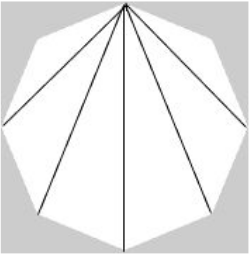So, we can construct 5*8 = 40 diagonals.
But we have constructed each diagonal twice, once from each of its ends. Thus there are 20 diagonals in a regular octagon.
 Question 33

If a connected graph G has planar embedding with 4 faces and 4 vertices, then what will be the number of edges in G?

 A 7 B 6 C 4 D 3
Engineering-Mathematics       Graph-Theory       JT(IT) 2018 PART-B Computer Science
Question 33 Explanation:
Euler's Formula for Planar Graphs:
For any(connected) planar graph with v vertices, e edges and faces, we have
V - E + F = 2
= 4 - E + 4 =2
E = 4 - 2 + 4
E = 6
 Question 34

Every cut set of a connected euler graph has____

 A An odd number of edges B At least three edges C An even number of edges D A single edge
Engineering-Mathematics       Graph-Theory       JT(IT) 2018 PART-B Computer Science
Question 34 Explanation:
If every minimal cut has an even number of edges, then in particular the degree of each vertex is even. Since the graph is connected, that means it is Eulerian.
 Question 35

A graph G is dual if and only if G is a ___

 A Euler graph B Regular graph C Complete graph D Planar graph
Engineering-Mathematics       Graph-Theory       JT(IT) 2018 PART-B Computer Science
Question 35 Explanation:
Deﬁnition Given a plane graph G, the dual graph G* is the plane graph whose vtcs are the faces of G.
→ The correspondence between edges of G and those of G* is as follows:
if e∈E(G) lies on the boundaries of faces X and Y, then the endpts of the dual edge e*∈(G*) are the vertices x and y that represent faces X and Y of G.Question 36

What is the chromatic number of an n-vertex simple connected graph, which does NOT contain any odd-length cycle?

 A N B N-1 C 2 D 3
Engineering-Mathematics       Graph-Theory       JT(IT) 2018 PART-B Computer Science
Question 36 Explanation:
→ If n ≥ 2 and there are no odd length cycles, Then it is bipartite graph.
→ A bipartite graph has the chromatic number 2.
Eg: Consider a square, which has 4 edges. It can be represented as bipartite ,with chromatic number 2.
 Question 37

Considering an undirected graph, which of the following statements is/are true?

(i) Number of vertices of odd degree is always even
(ii) Sum of degrees of all the vertices is always even
 A Neither (i) nor (ii) B Both (i) and (ii) C Only (ii) D Only (i)
Engineering-Mathematics       Graph-Theory       JT(IT) 2018 PART-B Computer Science
Question 37 Explanation:
True: Number of vertices of odd degree is always even.
True: Sum of degrees of all the vertices is always even.
 Question 38

Select the option that best describes the relationship between the following graphs: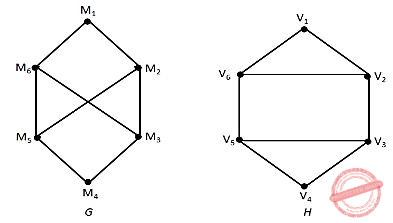A G and H are directed B G and H are isomorphic C G and H are homomorphic D G and H are not isomorphic
Engineering-Mathematics       Graph-Theory       JT(IT) 2018 PART-B Computer Science
Question 38 Explanation:
If G1 ≡ G2 then
1. |V(G1)| = |V(G2)|
2. |E(G1)| = |E(G2)|
3. Degree sequences of G1 and G2 are same.
4. If the vertices {V1, V2, ... Vk} form a cycle of length K in G1, then the vertices {f(V1), f(V2), … f(Vk)} should form a cycle of length K in G2.
→ Graph G and H are having same number of vertices and edges. So, it satisfied first rule.
→ u1 degree 2, u2 degree 3, u3 degree 3, u4 degree 2, u5 degree 3, u6 degree 3.
→ V1 degree 2, V2 degree 3, V3 degree 3, V4 degree 2, V5 degree 3, V6 degree 3.
→ But it violates the property of number of cycles. The number of cycles are not same.
 Question 39
The number of the edges in a regular graph of degree 'd' and 'n' vertices is____
 A Maximum of n,d B n+d C nd D nd/2
Engineering-Mathematics       Graph-Theory       Nielit Scientific Assistance CS 15-10-2017
Question 39 Explanation:
Sum of degree of vertices = 2*no. of edges
d*n = 2*|E|
∴ |E| = (d*n)/2
 Question 40

A minimal subgraph G’ of G such that V(G’)=V(G) and G’ is connected is called:

 A A spanning tree B A connected graph C A directed graph D A biconnected component
Engineering-Mathematics       Graph-Theory       JT(IT) 2016 PART-B Computer Science
Question 40 Explanation:
Given a connected graph G, a connected subgraph that is both a tree and contains all the vertices of G is called a spanning tree for G.
 Question 41
Consider a Hamiltonian Graph G with no loops or parallel edges and with |V(G)| = n ≥ 3. Then which of the following is true ?
 A deg(v) ≥n/2 for each vertex v. B |E(G)| ≥1/2(n – 1) (n – 2) + 2 C deg (v) + deg(w) ≥ n whenever v and w are not connected by an edge D All of the above
Engineering-Mathematics       Graph-Theory       UGC NET CS 2017 Jan -paper-2
Question 41 Explanation:
With the help of dirac’s theorem, we can prove above three statements.
 Question 42
Given the following graphs:Which of the following is correct?
 A G​ 1​ contains Euler circuit and G​ 2​ does not contain Euler circuit. B G​ 1​ does not contain Euler circuit and G​ 2​ contains Euler circuit. C Both G​ 1​ and G​ 2​ do not contain Euler circuit. D Both G​ 1​ and G​ 2​ contain Euler circuit.
Engineering-Mathematics       Graph-Theory       UGC NET CS 2016 Aug- paper-2
Question 42 Explanation:Step-1: G1 have odd number of vertices. So, it is not euler circuit.
Step-2: G2 also have odd number of vertices. So, it not euler circuit.
 Question 43
The number of different spanning trees in complete graph, K​ 4​ and bipartite graph, K​ 2,2​ have ______ and _______ respectively.
 A 14, 14 B 16, 14 C 16, 4 D 14, 4
Engineering-Mathematics       Graph-Theory       UGC NET CS 2016 July- paper-2
Question 43 Explanation:
Step-1: Given complete graph K​ 4​ .To find total number of spanning tree in complete graph using standard formula is n​ (n-2) Here, n=4
=n​ (n-2)
= 4​ 2
=16
Step-2: Given Bipartite graph K​ 2,2​ . To find number of spanning tree in a bipartite graph K​ m,n​ having standard formula is m​ (n-1)​ * n​ (m-1)​ .
m=2 and n=2
= 2​ (2-1)​ * 2​ (2-1)
= 2 * 2
= 4
 Question 44
A clique in a simple undirected graph is a complete subgraph that is not contained in any larger complete subgraph. How many cliques are there in the graph shown below?A 2 B 4 C 5 D 6
Engineering-Mathematics       Graph-Theory       UGC NET CS 2016 July- paper-2
Question 44 Explanation:
Definition of clique is already given in question.
Definition: A clique in a simple undirected graph is a complete subgraph that is not contained in any larger complete subgraph.
Step-1: b,c,e,f is complete graph.Step-2: ‘a’ is not connected to ‘e’ and ‘b’ is not connected to ‘d’. So, it is not complete graph.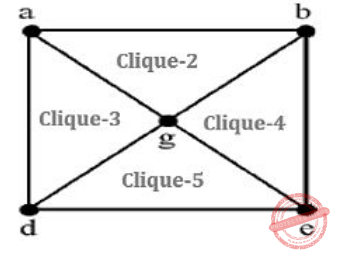Question 45
Which of the following statement(s) is/are false ?
(a) A connected multigraph has an Euler Circuit if and only if each of its vertices has even degree.
(b) A connected multigraph has an Euler Path but not an Euler Circuit if and only if it has exactly two vertices of odd degree.
(c) A complete graph (K​ n​ ) has a Hamilton Circuit whenever n ≥ 3.
(d) A cycle over six vertices (C​ 6​ ) is not a bipartite graph but a complete graph over 3 vertices is bipartite.
 A (a) only B (b) and (c) C (c) only D (d) only
Engineering-Mathematics       Graph-Theory       UGC NET CS 2015 Dec- paper-2
Question 45 Explanation:
(a)TRUE: A connected multigraph has an Euler Circuit if and only if each of its vertices has even degree.
(b)TRUE: A connected multigraph has an Euler Path but not an Euler Circuit if and only if it has exactly two vertices of odd degree.
(c)TRUE: A complete graph (K​ n​ ) has a Hamilton Circuit whenever n ≥ 3. (d) FALSE: A cycle over six vertices (C​ 6​ ) is not a bipartite graph but a complete graph over 3 vertices is bipartite.
 Question 46Consider the graph given below: The two distinct sets of vertices, which make the graph bipartite are:
 A (v​ 1​ , v​ 4​ , v​ 6​ ); (v​ 2​ , v​ 3​ , v​ 5​ , v​ 7​ , v​ 8​ ) B (v​ 1​ , v​ 7​ , v​ 8​ ); (v​ 2​ , v​ 3​ , v​ 5​ , v​ 6​ ) C (v​ 1​ , v​ 4​ , v​ 6​ , v​ 7​ ); (v​ 2​ , v​ 3​ , v​ 5​ , v​ 8​ ) D (v​ 1​ , v​ 4​ , v​ 6​ , v​ 7​ , v​ 8​ ); (v​ 2​ , v​ 3​ , v​ 5​ )
Engineering-Mathematics       Graph-Theory       UGC NET CS 2015 Dec- paper-2
Question 46 Explanation:
A bipartite graph (or bigraph) is a graph whose vertices can be divided into two disjoint and independent sets U and V such that every edge connects a vertex in U to one in V. Vertex sets U and V are usually called the parts of the graph. Equivalently, a bipartite graph is a graph that does not contain any odd-length cycles.
→ The two sets U and V may be thought of as a coloring of the graph with two colors.
Option A: FALSE because V​ 5​ , V​ 7​ and V​ 3​ are adjacent. So, it not not bipartite graph.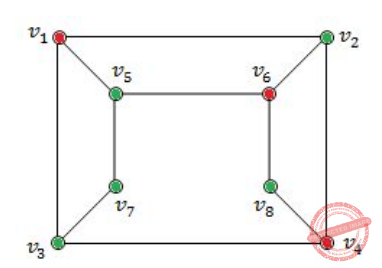Option-B FALSE because V​ 5​ , V​ 6​ and V​ 2​ are adjacent. So, it not not bipartite graph.Option-C TRUE because it follows properties of bipartied and no two colours are adjacent.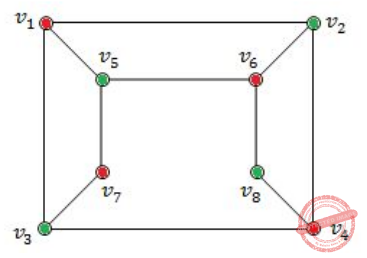Option-D FALSE because because V​ 4​ , V​ 6​ and V​ 8​ are adjacent. So, it not not bipartite graph.Question 47
A tree with n vertices is called graceful, if its vertices can be labelled with integers 1, 2,....n such that the absolute value of the difference of the labels of adjacent vertices are all different. Which of the following trees are graceful?
(a)(b)(c)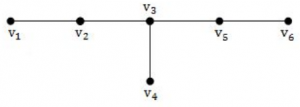A (a) and (b) B (b) and (c) C (a) and (c) D (a), (b) and (c)
Engineering-Mathematics       Graph-Theory       UGC NET CS 2015 Dec- paper-2
Question 47 Explanation:
Above all graphs are graceful.Question 48
Consider a Hamiltonian Graph (G) with no loops and parallel edges. Which of the following is true with respect to this Graph (G) ?
(a) deg (v) ≥ n / 2 for each vertex of G
(b) |E(G)| ≥ 1 / 2 (n - 1) (n - 2) + 2 edges
(c) deg (v) + deg (w) ≥ n for every n and v not connected by an edge.
 A (a) and (b) B (b) and (c) C (a) and (c) D (a), (b) and (c)
Engineering-Mathematics       Graph-Theory       UGC NET CS 2015 Jun- paper-2
Question 48 Explanation:
→ According to Dirac's theorem on Hamiltonian cycles, the statement that an n-vertex graph in which each vertex has degree at least n/2 must have a Hamiltonian cycle.
→ According to ore’s theorem,Let G be a (finite and simple) graph with n ≥ 3 vertices. We denote by deg v the degree of a vertex v in G, i.e. the number of incident edges in G to v. Then, Ore's theorem states that if deg v + deg w ≥ n for every pair of distinct non adjacent vertices v and w of G, then G is Hamiltonian.
→ A complete graph G of n vertices has n(n−1)/2 edges and a Hamiltonian cycle in G contains n edges. Therefore the number of edge-disjoint Hamiltonian cycles in G cannot exceed (n − 1)/2. When n is odd, we show there are (n − 1)/2 edge-disjoint Hamiltonian cycles.
So the statement(b) is false and statement a and c are true.
 Question 49
The following lists are the degrees of all the vertices of a graph :
(i) 1, 2, 3, 4, 5
(ii) 3, 4, 5, 6, 7
(iii) 1, 4, 5, 8, 6
(iv) 3, 4, 5, 6
then
 A (i) and (ii) B (iii) and (iv) C (iii) and (ii) D (ii) and (iv)
Engineering-Mathematics       Graph-Theory       UGC NET CS 2004 Dec-Paper-2
Question 49 Explanation:
Every graph is following basic 2 properties:
1. Sum of degrees of the vertices of a graph should be even.
2. Sum of degrees of the vertices of a graph is equal to twice the number of edges.
Statement-(i) is violating property-1.
= 1+2+3+4+5
= 15 is odd number.
Statement-(ii) is violating property-1.
= 3+4+5+6+7
= 25 is odd number.
Statement-(iii) is violating property-1.
= 1+4+5+8+6
= 24 is even number
Statement-(iv) is violating property-1
= 3+4+5+6
= 18 is even number
 Question 50
T is a graph with n vertices. T is connected and has exactly n-1 edges, then :
 A T is a tree B T contains no cycles C Every pairs of vertices in T is connected by exactly one path D All of these
Engineering-Mathematics       Graph-Theory       UGC NET CS 2005 Dec-Paper-2
Question 50 Explanation:
This is little bit tricky question.
Step-1:
n= number of vertices
n-1 = number of edges
Example: n=5 vertices and n-1=4 edgesStep-2: The above graph T won’t have cycle then we are calling as tree. Here, every pairs of vertices in T is connected by exactly one path.
Note: The above properties is nothing but minimum spanning tree properties.
 Question 51
The number of edges in a complete graph with N vertices is equal to :
 A N (N−1) B 2N−1 C N−1 D N(N−1)/2
Engineering-Mathematics       Graph-Theory       UGC NET CS 2006 Dec-paper-2
Question 51 Explanation:
N =5Question 52
For a complete graph with N vertices, the total number of spanning trees is given by :
 A 2N-1 B N​ N-1 C N​ N-2 D 2N+1
Engineering-Mathematics       Graph-Theory       UGC NET CS 2006 June-Paper-2
Question 52 Explanation:
If a graph is complete, total number of spanning trees are N​ N-2
Example:Formula to find total number of spanning trees are N​ N-2
=5​ 5-2
=5​ 3
=125
 Question 53
A certain tree has two vertices of degree 4, one vertex of degree 3 and one vertex of degree 2. If the other vertices have degree 1, how many vertices are there in the graph ?
 A 5 B n-3 C 20 D 11
Engineering-Mathematics       Graph-Theory       UGC NET CS 2014 Dec-Paper-2
Question 53 Explanation:
Method-1:
Here, they are clearly mentioned that “certain tree”
The tree contain maximum n-1 edges. Here, ‘n’ is number of vertices.
→ According to the handshaking lemma “sum of degrees of all vertices=2|e|”.
→ Two vertices of degree 4, we can write into (2*4)
→ One vertex of degree 3, we can write into (1*3)
→ One vertex of degree 2, we can write into (1*2).
→ Other vertices(X) have degree 1, we can write into (X*1)
Step-1: (2*4)+(1*3)+(1*2)+(X*1)
=2(X+4-1) [Note: ‘X’ value is not given ]
X=7
Step-2: To find total number of vertices, we are adding X+4 because they already given 4 vertices.
=X+4
=7+4
=11
Method-2:
Draw according to given constraints but it not suitable for very big treesB, C, E, F, H, I, J degree = 1
D degree = 2
A degree = 4
K degree = 4
G degree = 3
 Question 54
Consider the Graph shown below :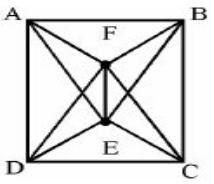This graph is a __________.
 A Complete Graph B Bipartite Graph C Hamiltonian Graph D All of the above
Engineering-Mathematics       Graph-Theory       UGC NET CS 2014 Dec-Paper-2
Question 54 Explanation:
Option-A: It is not complete graph because it won’t have n(n-1)/2 edges.
Option-B: It is not Bipartite Graph because it takes more than 2 colours. Bipartite Graph have exactly 2 colours.
Option-C: Hamiltonian path is a path in an undirected or directed graph that visits each vertex exactly once.Hence, Option-C is correct answer.
 Question 55
Consider the following statements related to AND-OR Search algorithm.
S​ 1​ : A solution is a subtree that has a goal node at every leaf.
S​ 2​ : OR nodes are analogous to the branching in a deterministic environment
S​ 3​ : AND nodes are analogous to the branching in a non-deterministic environment.
Which one of the following is true referencing the above statements?
Choose the correct answer from the code given below:
 A S1- False, S2- True, S3- True B S1- True, S2- True, S3- True C S1- False, S2- True, S3- False D S1- True, S2- True, S3- False
Engineering-Mathematics       Graph-Theory       UGC NET CS 2018-DEC Paper-2
Question 55 Explanation:
● An and–or tree is a graphical representation of the reduction of problems (or goals) to conjunctions and disjunctions of subproblems (or subgoals).
● A solution in an AND-OR tree is a sub tree whose leaves are included in the goal set
 Question 56
The K-coloring of an undirected graph G=(V,E) is a function C: V➝{0,1,......,K-1} such that c(u)≠c(v) for every edge (u,v) ∈ E Which of the following is not correct?
 A G has no cycles of odd length B G has cycle of odd length C G is 2-colorable D G is bipartite
Engineering-Mathematics       Graph-Theory       UGC NET CS 2018-DEC Paper-2
Question 56 Explanation:
● A k-colouring of a graph G consists of k different colours and G is then called k-colourable.
● A cycle of length n ≥ 3 is 2-chromatic if n is even and 3-chromatic if n is odd.
● A graph is bi-colourable (2-chromatic) if and only if it has no odd cycles.
● A nonempty graph G is bicolourable if and only if G is bipartite
 Question 57
​If a graph (G) has no loops or parallel edges and if the number of vertices(n) in the graph is n≥3, then the graph G is Hamiltonian if
(i) deg(v) ≥n/3 for each vertex v
(ii) deg(v) + deg(w) ≥ n whenever v and w are not connected by an edge.
(iii) E (G) ≥ 1/3 (n − 1 )(n − 2 ) + 2
 A (i) and (iii) only B (ii) and (iii) only C (iii) only D (ii) only
Engineering-Mathematics       Graph-Theory       UGC NET CS 2018-DEC Paper-2
Question 57 Explanation:
→ Dirac's theorem on Hamiltonian cycles, the statement that an n-vertex graph in which each vertex has degree at least n/2 must have a Hamiltonian cycle
→ Dirac's theorem on chordal graphs, the characterization of chordal graphs as graphs in which all minimal separators are cliques
→ Dirac's theorem on cycles in k-connected graphs, the result that for every set of k vertices in a k-vertex-connected graph there exists a cycle that passes through all the vertices in the set
 Question 58
Consider the graph given below as :Which one of the following graph is isomorphic to the above graph ?
 ABCDEngineering-Mathematics       Graph-Theory       UGC NET CS 2014 June-paper-2
Question 58 Explanation:
If two graphs are isomorphic, they must have:
→The same number of vertices, edges and degrees for corresponding vertices.
→The same number of connected components,loops and parallel edges.
The above graph each vertex having degree 3 and total number of vertices are 8. Total number of edges are 12.
Option-A: Vertex v5 having degree 4. So, it is not isomorphic graph.
Option-B: Vertex v6 having degree 4. So, it is not isomorphic graph.
Option-C: Every vertex having degree 3 and total number of edges and vertices are 12 and 8.
So, it is isomorphic.
Option-D: Vertex v6 having degree 4. So, it is not isomorphic graph.
 Question 59
Consider a complete bipartite graph km,n. For which values of m and n does this, complete graph have a Hamilton circuit
 A m = 3, n = 2 B m = 2, n = 3 C m = n ≥ 2 D m = n ≥ 3
Engineering-Mathematics       Graph-Theory       UGC NET CS 2014 June-paper-2
Question 59 Explanation:
Complete graph have a Hamilton circuit m=n ≥ 2. If G is connected simple graph with n vertices where n≥3, then G has a Hamilton circuit if the degree of each vertex is at least n/2.
 Question 60
Match List1 with List 2 and choose the correct answer from the code given below :Where V and E are the number of vertices and edges in graph respectively.
 A (a)-(i), (b)-(iii), (c)-(iv), (d)-(ii) B (a)-(i), (b)-(iii), (c)-(ii), (d)-(iv) C (a)-(iii), (b)-(i), (c)-(ii), (d)-(iv) D (a)-(iii), (b)-(i), (c)-(iv), (d)-(ii)
Regular-Expression       Graph-Theory       UGC NET CS 2018-DEC Paper-2
Question 60 Explanation:
→ Dijkstra's algorithm (or Dijkstra's Shortest Path First algorithm, SPF algorithm) is an algorithm for finding the shortest paths between nodes in a graph and time complexity is O(V​ 2​ )
→ Kruskal's algorithm is a minimum-spanning-tree algorithm which finds an edge of the least possible weight that connects any two trees in the forest and time complexity is O(E log E)
→ A topological sort or topological ordering of a directed graph is a linear ordering of its vertices such that for every directed edge uv from vertex u to vertex v,u comes before v in the ordering Θ(V + E)
→ Floyd–Warshall algorithm is an algorithm for finding shortest paths in a weighted graph with positive or negative edge weights. A single execution of the algorithm will find the lengths of shortest paths between all pairs of vertices
 Question 61
Consider an undirected graph G with 100 nodes. The maximum number of edges to be included in G so that the graph is not connected is
 A 2451 B 4950 C 4851 D 9900
Engineering-Mathematics       Graph-Theory       UGC NET CS 2013 Sep-paper-2
Question 61 Explanation:
Given data,
-- Undirected graph G with 100 nodes.
-- Maximum number of edges to be included in G so that the graph is not connected is ?
Step-1: As per the above description, it is simple undirected graph.
For simple graph using formula standard formula is ((n-1)*(n-2))/2
Step-2: Here, n=100
n-1=99
n-2=98
=((n-1)(n-2))/2
= (99*98)/2
= 4851
Note: The simple graph won’t have parallel edges and self loops.
 Question 62
Consider the following statements :
(i) A graph in which there is a unique path between every pair of vertices is a tree.
(ii) A connected graph with e = v – 1 is a tree.
(iii) A graph with e = v – 1 that has no circuit is a tree.
Which of the above statements is/are true ?
 A (i) & (iii) B (ii) & (iii) C (i) & (ii) D All of the above
Engineering-Mathematics       Graph-Theory       UGC NET CS 2013 Sep-paper-2
Question 62 Explanation:

Tree properties:

→A graph in which there is a unique path between every pair of vertices is a tree.
→A connected graph with e = v – 1 is a tree.
→A graph with e = v – 1 that has no circuit is a tree.
 Question 63
A simple graph G with n-vertices is connected if the graph has
 A (n – 1) (n – 2)/2 edges B more than (n – 1) (n – 2)/2 edges C less than (n – 1) (n – 2)/2 edges D Σki=1 C(ni, 2) edges
Engineering-Mathematics       Graph-Theory       UGC NET CS 2013 Sep-paper-2
Question 63 Explanation:
The simple graph won’t have parallel edges and self loops.
Simple graph properties:
→If a graph is connected then e≥n-1.
→If a graph has more than e>((n-1)(n-2)) / 2 then it is connected.
→The n vertex graph with the maximal number of edges that is still disconnected is a Kn-1
→A complete graph Kn-1 with n-1 vertices ((n-1)(n-2)) / 2 edges.
→Adding any possible edge must connect the graph, so the minimum number of edges needed to guarantee connectivity for an ‘n’ vertex graph is (((n−1)(n−2)) / 2)+1
 Question 64
A graph is non-planar if and only if it contains a subgraph homomorphic to
 A K3, 2 or K5 B K3, 3 and K6 C K3, 3 or K5 D K2, 3 and K5
Engineering-Mathematics       Graph-Theory       UGC NET CS 2013 Dec-paper-2
Question 64 Explanation:
A graph is non-planar if and only if it contains a subgraph which is homomorphic to k5 or k3,3. This is kuratowshi theorem.
 Question 65
The number of colours required to properly colour the vertices of every planar graph is
 A 2 B 3 C 4 D 5
Engineering-Mathematics       Graph-Theory       UGC NET CS 2012 June-Paper2
Question 65 Explanation:
→ The 4-colour theorem of the planar graph describes that any planar can at most be colored with 4 colors.
→ The sufficient number of colors in worst case is 4 colors for any planar graph.
 Question 66
Maximum number of edges in a n-Node undirected graph without self loop is
 A n2 B n(n-1) C n(n+1) D n(n-1)/2
Engineering-Mathematics       Graph-Theory       UGC NET CS 2011 Dec-Paper-2
Question 66 Explanation:
The set of vertices has size n, the number of such subsets is given by the binomial coefficient C(n,2)⋅C(n,2) = n(n-1)/2
 Question 67
Cyclometric complexity of a flow graph G with n vertices and e edges is
 A V(G) = e+n–2 B V(G) = e–n+2 C V(G) = e+n+2 D V(G) = e–n–2
Engineering-Mathematics       Graph-Theory       UGC NET CS 2013 June-paper-2
Question 67 Explanation:
Cyclomatic complexity uses 3 formulas:
1. The number of regions corresponds to the cyclomatic complexity
2. V(G),Flow graph is defined as V(G)=E-N+2 where E is the number of flow graph edges, and N is the number of flow graph nodes.
3. V(G),Flow graph is defined as V(G)=P+1 where p is the number of predicate nodes contained in the flow graph G.
 Question 68
How many edges must be removed to produce the spanning forest of a graph with N vertices, M edges and C connected components ?
 A M+N–C B M–N–C C M–N+C D M+N+C
Engineering-Mathematics       Graph-Theory       UGC NET CS 2013 June-paper-2
Question 68 Explanation:
→ Spanning forest is nothing but a set of spanning trees, one for each connected component in the graph
→ In the spanning forest the components are represented by a set of edges, rather than a set of vertices. Any vertices in the graph that are entirely unconnected will not appear in the spanning forest.
→ In spanning forest, we will use multiple trees to represent a path through the graph.
→ Consider the below exampleNumber of components(C)=3
Number of vertices(N)=9
Number of Edges(M)=8
By removing two edges, we can make spanning forest in the above graph.
The number of edges can be removed to make spanning forest is M-N+C=8-9+3=2
So option C is correct.
 Question 69
An undirected graph possesses an eulerian circuit if and only if it is connected and its vertices are
 A all of even degree B all of odd degree C of any degree D even in number
Engineering-Mathematics       Graph-Theory       UGC NET CS 2010 Dec-Paper-2
Question 69 Explanation:
→ An undirected graph possesses an eulerian circuit if and only if it is connected and its vertices all of even degree.
→ Eulerian circuit (or) Euler tour in an undirected graph is a cycle that uses each edge exactly once.
 Question 70
The minimum number of edges in a connected graph with ‘n’ vertices is equal to
 A n(n – 1) B n(n–1)/2 C n2 D n – 1
Engineering-Mathematics       Graph-Theory       UGC NET CS 2010 Dec-Paper-2
Question 70 Explanation:Question 71
S1 : I teach algorithms and maths.
S2 : My professor teaches maths, electronics and computer science.
S3 : I have a student of maths.
S4 : Algorithm is a part of computer science.
S5 : Maths students know computer science.
What would be the chromatic number of a graph, vertices of which are the actors/entities that are involved in the sentences S1 to S5 and edges-to represent the associations/relationships amongst the entities/actors as expressed in the sentences S1 to S5 above ?
 A 2 B 3 C 4 D None of these
Engineering-Mathematics       Graph-Theory       UGC NET CS 2010 June-Paper-2
 Question 72
The complete graph with four vertices has k edges where k is :
 A 3 B 4 C 5 D 6
Engineering-Mathematics       Graph-Theory       UGC NET CS 2009-June-Paper-2
Question 72 Explanation:
To find number of edges in complete graph using standard formula is n(n-1)/2n=4
= 4(4-3)/2
= (4*3)/2
= 12/2
= 6
 Question 73
Which two of the following are equivalent for an undirected graph G ?
(i) G is a tree
(ii) There is at least one path between any two distinct vertices of G
(iii) G contains no cycles and has (n-1) edges
(iv) G has n edges
 A (i) and (ii) B (i) and (iii) C (i) and (iv) D (ii) and (iii)
Engineering-Mathematics       Graph-Theory       UGC NET CS 2009-June-Paper-2
Question 73 Explanation:
Option-A: FALSE because undirected graph will be cycle also.
Option-B: TRUE because there is at least one path between any two distinct vertices of G. otherwise we are no calling as undirected graph.
Option-C: TRUE. If G contains no cycles and has (n-1) edges.
Option-D: FALSE
 Question 74
The number of edges in a complete graph of n vertices is
 A n B n(n – 1)/2 C n(n + 1)/2 D n2/2
Engineering-Mathematics       Graph-Theory       UGC NET CS 2009 Dec-Paper-2
Question 74 Explanation:
Complete graph is an undirected graph in which each vertex is connected to every vertex, other than itself. If there are ‘n’ vertices then total edges in a complete graph is n(n-1)/2.
Ex:n=5 and complete graph
Using formula to find number of edges is n(n-1)/2
=5*(5-1)/2
=(5*4)/2
=10
 Question 75
The graph K3,4 has :
 A 3 edges B 4 edges C 7 edges D 12 edges
Engineering-Mathematics       Graph-Theory       UGC NET CS 2008 Dec-Paper-2
Question 75 Explanation:
The graph K3,4 means it complete bipartite graph with m=4 and n=3
Total number of vertices= n + m
Total number of edges= m*n
Example: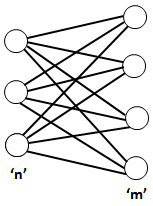Question 76
The number of edges in a complete graph with ‘n’ vertices is equal to :
 A n(n-1) B n(n-1)/2 C n2 D 2n-1
Engineering-Mathematics       Graph-Theory       UGC NET CS 2007-Dec-Paper-2
Question 76 Explanation:
Complete graph is an undirected graph in which each vertex is connected to every vertex, other than itself. If there are ‘n’ vertices then total edges in a complete graph is n(n-1)/2.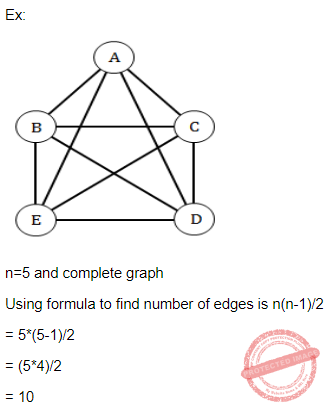There are 76 questions to complete.
PHP Code Snippets Powered By : XYZScripts.com